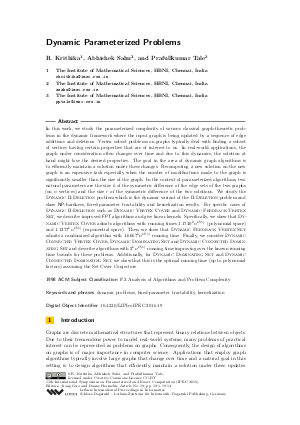Document# Dynamic Parameterized Problems

### Authors R. Krithika, Abhishek Sahu, Prafullkumar Tale## File

LIPIcs.IPEC.2016.19.pdf
• Filesize: 0.49 MB
• 14 pages

## Cite As

R. Krithika, Abhishek Sahu, and Prafullkumar Tale. Dynamic Parameterized Problems. In 11th International Symposium on Parameterized and Exact Computation (IPEC 2016). Leibniz International Proceedings in Informatics (LIPIcs), Volume 63, pp. 19:1-19:14, Schloss Dagstuhl - Leibniz-Zentrum für Informatik (2017)
https://doi.org/10.4230/LIPIcs.IPEC.2016.19

## Abstract

In this work, we study the parameterized complexity of various classical graph-theoretic problems in the dynamic framework where the input graph is being updated by a sequence of edge additions and deletions. Vertex subset problems on graphs typically deal with finding a subset of vertices having certain properties that are of interest to us. In real-world applications, the graph under consideration often changes over time and due to this dynamics, the solution at hand might lose the desired properties. The goal in the area of dynamic graph algorithms is to efficiently maintain a solution under these changes. Recomputing a new solution on the new graph is an expensive task especially when the number of modifications made to the graph is significantly smaller than the size of the graph. In the context of parameterized algorithms, two natural parameters are the size k of the symmetric difference of the edge sets of the two graphs (on n vertices) and the size r of the symmetric difference of the two solutions. We study the Dynamic Pi-Deletion problem which is the dynamic variant of the Pi-Deletion problem and show NP-hardness, fixed-parameter tractability and kernelization results. For specific cases of Dynamic Pi-Deletion such as Dynamic Vertex Cover and Dynamic Feedback Vertex Set, we describe improved FPT algorithms and give linear kernels. Specifically, we show that Dynamic Vertex Cover admits algorithms with running times 1.1740^k*n^{O(1)} (polynomial space) and 1.1277^k*n^{O(1)} (exponential space). Then, we show that Dynamic Feedback Vertex Set admits a randomized algorithm with 1.6667^k*n^{O(1)} running time. Finally, we consider Dynamic Connected Vertex Cover, Dynamic Dominating Set and Dynamic Connected Dominating Set and describe algorithms with 2^k*n^{O(1)} running time improving over the known running time bounds for these problems. Additionally, for Dynamic Dominating Set and Dynamic Connected Dominating Set, we show that this is the optimal running time (up to polynomial factors) assuming the Set Cover Conjecture.
##### Keywords
• dynamic problems
• fixed-parameter tractability
• kernelization

## Metrics

• Access Statistics
• Total Accesses (updated on a weekly basis)
0

## References

1. F. N. Abu-Khzam, J. Egan, M. R. Fellows, F. A. Rosamond, and P. Shaw. On the Parameterized Complexity of Dynamic Problems. Theoretical Computer Science, 607 (3):426-434, 2015.2. L. Cai. Fixed-Parameter Tractability of Graph Modification Problems for Hereditary Properties. Information Processing Letters, 58(4):171-176, 1996.3. J. Chen, I. A. Kanj, and W. Jia. Vertex Cover: Further Observations and Further Improvements. Journal of Algorithms, 41(2):280-301, 2001.4. J. Chen, I. A. Kanj, and G. Xia. Improved Upper Bounds for Vertex Cover. Theoretical Computer Science, 411(40-42):3736-3756, 2010.5. M. Cygan, H. Dell, D. Lokshtanov, D. Marx, J. Nederlof, Y. Okamoto, R. Paturi, S. Saurabh, and M. Wahlstrom. On Problems As Hard As CNF-SAT. In Proceedings of the IEEE Conference on Computational Complexity, CCC'12, pages 74-84. IEEE Computer Society, 2012.6. M. Cygan, F. V. Fomin, K. Łukasz, D. Lokshtanov, D. Marx, M. Pilipczuk, M. Pilipczuk, and S. Saurabh. Parameterized Algorithms. Springer, 2015.7. M. Cygan, J. Nederlof, M. Pilipczuk, M. Pilipczuk, J. M. M. van Rooij, and J. O. Wojtaszczyk. Solving Connectivity Problems Parameterized by Treewidth in Single Exponential Time. In Proceedings of the IEEE 52^nd Annual Symposium on Foundations of Computer Science, FOCS'11, pages 150-159, 2011.8. R. Diestel. Graph Theory. Springer-Verlag Berlin Heidelberg, 2006.9. R. G. Downey, J. Egan, M. R. Fellows, F. A. Rosamond, and P. Shaw. Dynamic Dominating Set and Turbo-Charging Greedy Heuristics. Tsinghua Science and Technology, 19(4):329-337, 2014.10. R. G. Downey and M. R. Fellows. Fundamentals of Parameterized Complexity. Springer-Verlag London, 2013.11. J. Flum and M. Grohe. Parameterized Complexity Theory. Springer, 2006.12. F. V. Fomin, S. Gaspers, D. Lokshtanov, and S. Saurabh. Exact Algorithms via Monotone Local Search. In Proceedings of the 48^th Annual ACM Symposium on Theory of Computing, STOC'16. ACM, 2016.13. F. V. Fomin, S. Gaspers, S. Saurabh, and A. A. Stepanov. On Two Techniques of Combining Branching and Treewidth. Algorithmica, 54(2):181-207, 2009.14. F. V. Fomin, D. Kratsch, and G. J. Woeginger. Exact (exponential) Algorithms for the Dominating Set Problem. In Proceedings of the 30^th International Workshop on Graph-Theoretic Concepts in Computer Science, WG'04, pages 245-256. Springer Berlin Heidelberg, 2004.15. S. Hartung and R. Niedermeier. Incremental List Coloring of Graphs, Parameterized by Conservation. Theoretical Computer Science, 494:86-98, 2013.16. S. Khot and V. Raman. Parameterized Complexity of Finding Subgraphs with Hereditary Properties. Theoretical Computer Science, 289(2):997-1008, 2002.17. J. Kneis, D. Mölle, S. Richter, and P. Rossmanith. A Bound on the Pathwidth of Sparse Graphs with Applications to Exact Algorithms. SIAM Journal on Discrete Mathematics, 23(1):407-427, 2009.18. T. Kociumaka and M. Pilipczuk. Faster Deterministic Feedback Vertex Set. Information Processing Letters, 114(10):556-560, 2014.19. J. M. Lewis and M. Yannakakis. The Node-Deletion Problem for Hereditary Properties is NP-Complete. Journal of Computer and System Sciences, 20(2):219-230, 1980.20. N. Misra, G. Philip, V. Raman, S. Saurabh, and S. Sikdar. FPT Algorithms for Connected Feedback Vertex Set. Journal of Combinatorial Optimization, 24(2):131-146, 2012.21. S. Thomassé. A Quadratic Kernel for Feedback Vertex Set. In Proceedings of the 12^th Annual ACM-SIAM Symposium on Discrete Algorithms, SODA'09, pages 115-119, 2009.22. M. Xiao and H. Nagamochi. Exact Algorithms for Maximum Independent Set. In Proceedings of the 24^th International Symposium on Algorithms and Computation, ISAAC'13, pages 328-338. Springer Berlin Heidelberg, 2013.X

Feedback for Dagstuhl Publishing